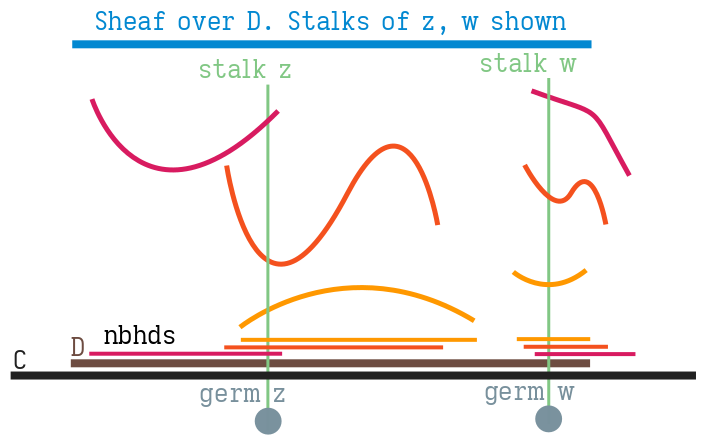## § Elementary uses of Sheaves in complex analysis

I always wanted to see sheaves in the wild in a setting that was both elementary but 'correct': In that, it's not some perverse example created to show sheaves (DaTaBaSeS arE ShEAvEs). Ahlfors has a great example of this which I'm condensing here for future reference.

#### § Sheafs: Trial 1

• We have function elements $(f: \Omega \rightarrow \mathbb C, \Omega \subseteq \mathbb C)$. $f$ is complex analytic, $\Omega$ is an open subset of $\mathbb C$.
• Two function elements $(f_1, \Omega_1), (f_2, \Omega_2)$ are said to be analytic continuations of each other iff $\Omega_1 \cap \Omega_2 \neq \emptyset$, and $f_1 = f_2$ on the set $\Omega_1 \cap \Omega_2)$.
• $(f_2, \Omega_2)$ can be called as the continuation of $(f_1, \Omega_1)$ to region $\Omega_2$.
• We will have that the analytic continuation of $f_1$ to $\Omega_2$ is unique. If there exists a function element $(g_2, \Omega_2)$, $(h_2, \Omega_2)$ such that $g_2 = f_1 = h_2$ in the region $\Omega_1 \cap \Omega_2$, then by analyticity, this agreement will extend to all of $\Omega_2$.
• Analytic continuation is therefore an equivalence relation (prove this!)
• A chain of analytic continuations is a sequence of $(f_i, \Omega_i)$ such that the adjacent elements of this sequence are analytic continuations of each other. $(f_i, \Omega_i)$ analytically continues $(f_{i+1}, \Omega_{i+1})$.
• Every equivalence class of this equivalence relation is called as a global analytic function. Put differently, it's a family of function elements $(f, U)$ and $(g, V)$ such that we can start from $(f, U)$ and build analytic continuations to get to $(g, V)$.

#### § Sheafs: Trial 2

• We can take a different view, with $(f, z \in \mathbb C)$ such that $f$is analytic at some open set $\Omega$ which contains $z$. So we should picture an $f$ sitting analytically on some open set $\Omega$ which contains $z$.
• Two pairs $(f, z)$, $(f', z')$ are considered equivalent if $z = z'$ and $f = f'$ is some neighbourhood of $z (= z')$.
• This is clearly an equivalence relation. The equivalence classes are called as germs .
• Each germ $(f, z)$ has a unique projection $z$. We denote a germ of $f$ with projection $z$as $f_z$.
• A function element $(f, \Omega)$ gives rise to germs $(f, z)$ for each $z \in \Omega$.
• Conversely, every germ $(f, z)$ is determined by some function element $(f, \Omega)$since we needed $f$ to be analytic around some open neighbourhood of $z$: Call this neighbourhood $\Omega$.
• Let $D \subseteq \mathbb C$ be an open set. The set of all germs $\{ f_z : z \in D \}$ is called as a sheaf over $D$. If we are considering analytic $f$ then this will be known as the sheaf of germs of analytic functions over $D$. This sheaf will be denoted as $Sh(D)$.
• There is a projection $\pi: Sh(D) \rightarrow D; (f, z) \mapsto z$. For a fixed $z0 \in D$, the inverse-image $\pi^{-1}(z0)$ is called as the stalk over $z0$. It is denoted by $Sh(z)$.
• $Sh$ carries both topological and algebraic structure. We can give the sheaf a topology to talk about about continuous mappings in and out of $Sh$. It also carries a pointwise algebraic structure at each stalk: we can add and subtract functions at each stalk; This makes it an abelain group.

#### § Sheaf: Trial 3

A sheaf over $D$ is a topological space $Sh$ and a mapping $\pi: Sh \rightarrow D$ with the properties:
• $\pi$ is a local homeomorphism. Each $s \in S$ has an open neighbourhood $D$such that $\pi(D)$ is open, and the restriction of $\pi$ to $D$ is a homeomorphism.
• For each point $z \in D$, the stalk $\pi^{-1}(z) \equiv S_z$ has the structre of an abelian group.
• The group operations are continuous with respect to the topology of $Sh$.
We will pick $D$ to be an open set in the complex plane; Really, $D$ can be arbitrary.

#### § Germs of analytic functions satisfy (Sheaf: Trial 3)